### Book on the subject: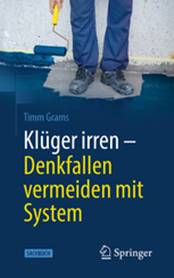In the table of mortality from tuberculosis in New York and Richmond from 1910 we encounter Simpson's paradox (Székely, 1990, pp. 63, 75 and 133):

 population Deaths Death rate new York Richmond new York Richmond new York Richmond White 4 675 174 80 895 8 365 131 0,00179 0,00162 Coloured 91 709 46 733 513 155 0,00560 0,00332 total 4 766 883 127 628 8 878 286 0,00186 0,00224

Contradiction. If you know, go to Richmond. If you are colored, go to Richmond as well. Are you white or colored, then stay in New York.

Analysis. You don't have to falsify statistics if you want to mislead. Sometimes it is enough to aggregate counting data, as here. The mechanism of this aggregation trap becomes somewhat more transparent in the xenophobia example. The study Simpson.pdf shows how one can overcome the paradox not only with formal, but also with graphic means.

(1998, 12.07.2010)

Back to overview

### Benford's Law

The mathematician Mark Nigrini wrote a program that can be used to track down fake tax returns. It takes advantage of the fact that in naturally occurring numbers the initial digits have a certain distinctive distribution. Fake numbers usually deviate significantly from this.

Every day we come across numbers: numbers that are on the front page of a daily newspaper, numbers about the size of inland waters in Germany, the number of inhabitants in cities or family incomes, the credit balances in a bank's accounts and the numbers on a tax return. When asked about the probability that any picked number starts with a 1, most people will answer, “The probability is 1/9.” They assume that all digits from 1 to 9 are equally probable (provided that they remembered that zeros are practically never at the beginning of numbers).

Contradiction. If you look at the distribution of the leading digits in the areas of the countries of the world, a different picture emerges: In about 30% of the cases the number starts with a one. A similar picture emerges with the number of inhabitants and with the gross national product per capita. There is a rule behind this: this applies to an astonishingly wide range Benfordsche law (Székely, 1990, p. 189).

The following graphic shows the distribution of the leading digits according to this law and, in comparison, the distributions of the leading digits of statistical key figures of the 192 independent countries of the world based on the Aktuell 2000 yearbook.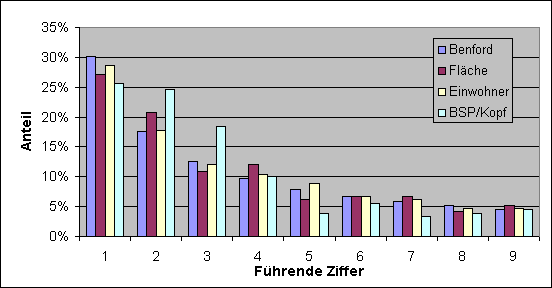Benford's Law. The probability p(d) for that a number with the digit d begins is given by p(d) = ln (1 + 1 /d) / ln (b). In here is b the basis of the place value system for the representation of numbers. In the decimal system is b = 10 and Benford's law simplifies to p(d) = log (1 + 1 /d). Log denotes the decadic logarithm.

Analysis. Benford's law describes an empirical fact. In the literature one can also find justifications. These make certain assumptions about the statistics and derive the law from them. I make the law clear to myself as follows: Many numerical phenomena are governed, at least temporarily, by exponential growth (or exponential shrinkage). An example is the growth of balances in a bank account (compound interest calculation). The number to be measured x so grows exponentially over time t. That means: The same percentage increase is always achieved in the same time periods. Until a leading 1 is replaced by a 2, the value x double. Until the 2 is replaced by the 3, the number only has to increase by 50%. The time required for this is correspondingly reduced. In general, the following applies: The numbers with a certain number of digits, those with the digit d begin, describe a value increase by the fraction 1 / d. A simple calculation shows that the xValues ​​with the number d in the first place on the time axis a proportion of ln (1 + 1 /d) / ln (b) turn off. If the process is encountered at a random point in time, the probability distribution for the first digit will therefore satisfy Benford's law.

If you are looking for further explanations, you can look up for example at Kevin Brown and Eric Weisstein.

(April 16, 1999, April 25, 2000)

Back to overview

### xenophobia

In the town Falldala with 20,000 inhabitants, the proportion of foreigners is 30%. The following table shows the crime statistics. From this it can be concluded that foreigners are more prone to crime than nationals.

#### Falldala crime statistics

Residents

Offenses per year

based on per 1000 inhabitants

Foreigners

6000

51

8.5

Resident

14000

59

4.2

Contradiction. A closer look at the crime statistics shows that in one part of the city - let's call it Aschental - crime is particularly high. Now, due to the unattractive development from the 50s for residents, mainly foreigners have settled in the Aschental. 5,000 of the total of 10,000 residents are foreigners. The rest of them live in the city center. The breakdown of the statistics looks like this:

#### Aschental crime statistics

Residents

Offenses per year

based on per 1000 inhabitants

Foreigners

5000

50

10

Resident

5000

50

10

#### Downtown Falldala crime statistics

Residents

Offenses per year

based on per 1000 inhabitants

Foreigners

1000

1

1

Resident

9000

9

1

It turns out that foreigners are no more or less likely to commit crimes than nationals. The initial presumption of foreigner crime turns out to be too superficial. A closer look suggests completely different interpretations: Perhaps it is due to the environment, the poverty, the district's milieu. In any case, more in-depth analyzes are required.

Analysis.:It is about a Aggregation trap like Simpson's Paradox. The aggregation of counting data leads to a narrowing of the view - the population is out of sight (headlight principle). The unspecific aggregation of counting data creates relationships that do not exist in the broken down statistics. Here there is a connection between the attributes “foreigner” and “tendency to crime”.

In addition, the statistical connection is inadmissibly interpreted in terms of a causal connection: a person who is a foreigner is more prone to criminal acts than a resident. That's a thought trap on the Causality expectation - an innate teacher (Konrad Lorenz) - goes back. The fact that many people believe in astrology could be due to the same pattern of thought, as can be seen from several articles in the spectrum of science (Michael Springer, 4/1999, p. 106; Gunter Sachs, 7/1999, p. 9; Herbert Basler, 8 / 1999, p. 94 ff.).

(6.9.1999)

Back to overview

The four cards shown contain a letter on one side and a number on the other.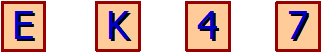Which cards do you have to turn over if you want to determine whether the following statement applies: "If there is a vowel on one side of the card, then there is an even number on the other side"?

Contradiction. In a psychological experiment, most of the test subjects chose the cards with the E and the one with the 4. There is no point in turning the card with the 4! Whatever letter is on the back: it matches the statement to be checked. Only by turning over the cards with the E and the 7 do we have a chance to refute the statement. Only a minority of 4% of respondents chose this option.

Analysis. Our facility for induction, i.e. the ability to draw extension conclusions and form theories, works according to the following argumentation pattern: If the theory (hypothesis) H an event E. predicts and if at the same time the event E. based on previous knowledge is quite unlikely, then the theory will H based on an observation of the event E. more believable. In short: from "H implies E." and "E. is true "follows"H becomes more credible ”. We tend to enrich this plausible conclusion (induction conclusion) with greater certainty: From "H implies E." and "E. is true "we mean on"H is true “to be able to conclude; but that is an impermissible reverse conclusion. This thought trap is also called Failure at the mode of Tollens (Anderson, 1988, p. 248). The induction thought trap even strikes twice in Wason's selection problem:

1.      Because the theory H by a correctly predicted event E. becomes more believable, we look for exactly such events. This addiction to confirmation is an inevitable companion of our induction facility. It tempts us to turn over the card with the 4 on it. Scientists in particular run the risk of succumbing to the addiction to confirmation: the theory they have put forward and loved is supposed to prove itself and not turn out to be useless or false.

2.      Theory H is itself formulated as an implication: From “there is a vowel on the card” follows “there is an even number on the card”. The often drawn but unauthorized reverse conclusion looks like this: From “There is an even number on the card” follows “There is a vowel on the card”. In this light, the selection of the card with the 4 is reasonable. Due to the unauthorized reverse conclusion, the theory appears to be stricter than it is. In this stricter version, it could even be refuted by the card with the K, for example if there were a 2 on the back.

If one were to prove a theory by corroboration, one would have to check all of its predictions - not just some of the correct ones. In the case of scientific theories with their far-reaching statements, this is a hopeless undertaking. In contrast, theories can be refuted (falsified) by showing a single counterexample. In our case, only the card with the E and the card with the 7 offer this chance. Even if we are not looking for rebuttals: we will quickly see them. We behave like “passive Popperians,” says Evans (1989).

(05.11.05)

Back to overview

### The Harvard Medical School Study

We are looking at a test for a disease that has a base rate of 1/1000 - that is, one in a thousand people is sick. The test gives a wrong result with a probability of 5%. In particular, it has a false positive rate of 5%. What is the likelihood that a person who tests positive will actually have the disease?

Contradiction. The most common judgment from professors, doctors and students is "95%". However, the analysis shows that the probability is actually below 2%. Let the following bar represent a thousand people. Of the thousand people (on average) one is really sick. It is represented by the dark field on the far left. The test is false positive in around 50 people. Of the 51 people with a positive test, only one is really sick, which corresponds to a proportion of less than 2% (Hell / Fiedler / Gigerenzer, 1993).

Analysis: The fallacy observed here is a stochastic variant of logical fallacies of the following kind: From the sentences "When it rains the earth gets wet" and "The earth is wet" we infer - wrongly - on "It rained". That is the occasional one Failure at the mode of Tollens (Anderson, 1988, p. 248 ff.). So we infer the cause from the effect. But at best we can view the cause as more plausible based on the observed effect - but by no means as compelling. In the Harvard Medical School study, it is the disease that we see as the cause of the positive test result. Here, too, an at best plausible conclusion is overinterpreted as imperative. The systematic and blatant misjudgment is based on an overestimation of confirmatory information.

If you want to read more about statistical errors of all kinds and how they are “overwhelmed”, you will find it in the book by Beck-Bornholdt and Dubben (2001).

(22.12.1999)

Back to overview

### Software verification and testing

In a textbook on the subject of reliability verification of software I find the sentence highlighted in bold: “The Test of hypotheses goes through the falsification of their complement ”(Ehrenberger, 2002, p. 116). It is then shown that in the event of a situation that does not correspond to the hypothesis, the test will, in the worst case, pass the test with a low probability (say 5%). From the passing of the test it is concluded that the complement is unlikely (5%) and the hypothesis is correspondingly probable (95%).

Contradiction. There are two mistakes in the "proof". Firstly, a negative test result (“passed”) does not falsify the complement of the hypothesis, but rather a very specific fact, namely the alternative hypothesis. Refutation of the alternative hypothesis alone does not show that the hypothesis is true. Second, a probability statement about test results is taken as the probability statement about the hypotheses. And there is no justification for this. When calculating the probabilities, the alternative hypothesis was assumed to be firmly given. The probabilities are therefore conditional probabilities. The thought structure lacks the mathematical-logical foundation, it is built on sand.

Analysis (with a little more math than usual). Thinking mechanisms that already played a role in Wason's selection exercise and in the Harvard Medical School study contribute to the errors. To make things clearer, I will follow up on the mathematical notation already introduced there. In test theory, the original hypothesis is called the null hypothesis, for short H0. I denote the complement here with ¬H0 (spoken: “not H zero”). It is either H0or ¬H0. The alternative hypothesis H1 is a certain state of affairs incompatible with the hypothesis. The following applies: H1 implies ¬H0. The first fallacy goes back to our appendix to induction, to the plausible inference instead of the exact deduction required here: From the refutation of the alternative hypothesis, from ¬H1 so, one thinks onH0 to be able to close. That is a mistake. Now to the second fallacy: E. denote the observation that the test passed. The starting point of the "proof" is the determination that the test will be passed with a probability of only 5% if the alternative hypothesis is present: p(E. |H1) = 5%. From this it is concluded that the alternative hypothesis can be true with only 5 percent probability if the test is passed: p(H|E.) = 5%. That is an unauthorized reverse conclusion, another induction error. Although the probability canp(H|E.) using the Bayesian formula. At the latest in the course of the calculation, it becomes apparent that additional data is required. You need the a priori probabilities of the hypotheses before you can say anything about the effects of the test. And such a prioris is nowhere mentioned in the “proof” mentioned. (On the background of this example: The engineer's difficulties with the inferring statistics.)

Additive. Paradoxically, poorly founded theories are not exactly rare in quality assurance. I recently discovered an example: the "exact" formulas of Clopper and Pearson. These formulas for estimating a probability are repeatedly referred to in textbooks, along with a rather strange “derivation”. It has long been known that the formulas are anything but exact. In the script “Fundamentals of Quality and Risk Management” I criticize some of the questionable “theories” from the world of reliability and security: Bayes estimation versus test theory, X-Ware reliability, diverse programming (software diversity), reliability growth models.

(29.05.08)

Back to overview

### Bayesian estimate

In the section on the Harvard Medical School study, a simple frequency analysis is used to estimate the effectiveness of a diagnostic test. The transition from frequencies to probabilities results in the Bayes estimate (Sachs, 1992). What is so successfully applied to diagnostic tests should be transferable to parameter estimates. It is no longer a matter of estimating whether a certain disease is present or not, but rather of estimating an influencing variable (parameter) that cannot be directly measured.An example of such a parameter is the error probability of a product that is mass-produced under certain conditions. The probability of error is unknown. But you can get a sample of the product and determine the proportion of defective copies. As with the diagnostic test, a probability statement about the actually interesting facts (illness or error probability) should be derived from the observed result (test result or error percentage). In both cases it is about the probabilities of hypotheses, on the one hand about hypotheses about a disease and the other time about hypotheses about possible error probabilities. In both cases an initial estimate is needed. In the diagnostic test, this is the base rate of the disease. The parameter estimation is based on a more or less arbitrary estimation of the hypothesis probabilities (a priori probabilities). By applying Bayes’s formula, this estimate is improved, taking into account the observation made (a posteriori probabilities).

Contradiction. Applying Bayes' formula to parameter estimates can lead to paradoxical results. There are cases where the estimate gets worse the more data you take into account.

Analysis. At its core, Bayes’s formula says that the probability of a hypothesis H (“Person is sick”) through the observation made E. (“Test is positive”) increases in the same proportion as the observation by the hypothesis becomes more probable. With the formula symbols for (conditional) probabilities, this relationship looks like this: P(H|E.)/P(H) = P(E.|H)/P(E.). In the case of the Harvard Medical School study, we assume that the following data are known: P(H)=0,1%, P(E.|H) = 95% (sensitivity of the test), P(E.) ≈5.1%. The formula then provides the result: A person who tested positive is actually ill with a probability of less than 2%. This application of Bayes' formula to diagnostic tests is methodologically flawless. The probabilities P(H) and P(H|E.) are the a priori and a posteriori probability of the hypothesis.

The fact that the parameter estimation sometimes does not work properly is attributed to two causes in the literature (Fisz, 1976, p. 580; Papoulis, 1965, p. 114):

1.      An initial estimate of the distribution of the parameter is needed. And mostly nothing is known about this a priori; it is set arbitrarily.

2.      Since the parameter to be estimated is generally an unknown but at least fixed variable, it should not actually be viewed as a random variable. The conclusions from Bayes' formula are impractical in this sense.

From a mathematical point of view, these objections are rather harmless. You alone cannot explain the paradoxical results. I see another reason for the occasional malfunction of the parameter estimation with Bayes' method.

3.      The a posteriori distribution of the parameter is sometimes misinterpreted. It is - compared to the a priori distribution - as a better estimate of the actual distribution of the parameter. The a posteriori distribution is only an improved estimate on condition of the observation made. In other words: the distribution indicates the probabilities with which the various parameter values ​​could have contributed to the result observed. The reverse conclusion from the observation to the unconditional hypothesis is at best plausible, it is an induction conclusion. Failure occurs when too strong conclusions are drawn from an observation.

In medical diagnostics, the a posteriori probability is actually not taken as an improved probability estimate compared to the a priori: the base rate of the disease remains unchanged. The calculated a posteriori probability of illness only applies to people with a positive test result and not to “the whole world”. Let me give you a simple example to illustrate this third point.

 Table of the probabilities of all combinations Payout E (Measurement result) Hypotheses about the Number of coins in the game Line total H1 H2 H3 0 4/24 2/24 1/24 7/24 1 4/24 4/24 3/24 11/24 2 0 2/24 3/24 5/24 3 0 0 1/24 1/24 Column total 1/3 1/3 1/3 1

The one euro game.You are offered to take part in the following game for a euro stake. In secret, one, two or three coins are selected by random selection and then thrown. For every coin that shows heads, one euro is paid out. They want to know if the game is worth it for you. From the probabilities of choosing one, two or three coins, and assuming that the coins are fair (50% probability each for heads and tails), you want to determine the profit expectation. The trouble is: You don't know the distribution of the parameter “number of coins”. You watch the game for a while and want to find the best possible estimate of this distribution from the observed payouts and with the help of Bayesian formula. You name the hypotheses that 1, 2 or 3 coins are tossed, H1, H2 and H3. As an initial estimate, assume - according to the principle of indifference - the uniform distribution: P(H1) = P(H2) = P(H3) = 1/3. In a table you put together the resulting probabilities for all possible events (combinations of coin number and payout).

Now do the observation E.: One euro is paid out. Bayesian formula gives you the posterior probabilities of the hypotheses:

P(H1|E.) = P(H1)∙P(E.|H1)/P(E.) = P(EH1)/P(E.) = (4/24)/(11/24) = 4/11,

P(H2|E.) = 4/11,

P(H3|E.) = 3/11.

Taking into account the initial distribution of the parameter, this leads to the conclusion that there was only one coin involved in the specific game result (namely one euro payment) with a probability of 4/11; with the same probability it was two coins and with the probability 3/11 it was three coins. Correct your estimate of the hypothesis probabilities accordingly.

Be warned. The reassessment of the hypotheses using the a posteriori probabilities can sometimes be justified from a pragmatic point of view, but it is not mathematically justifiable. And it can produce absurd results. And that's the case in this game. For example, a payout of three euros would lead to the final assessment that there are basically three coins in play. Then you would be trapped and run the risk of overestimating the profit expectations.

The situation is different if the "slot machine" always stays with its coin selection for a whole day - that is, over many games. In this case, your Bayesian estimate will work and you can then decide for the day whether or not to participate.

(10.09.2008)

Back to overview

Two envelopes contain money, one twice as much as the other. I can choose an envelope and take the money out. Then I can decide whether I want to keep the money or switch to the other envelope. Assuming I pull out an envelope and find € 100 in it, a brief reflection shows me that I should accept the offer to exchange it: Since I chose the envelope purely at random, the probability that I first took the smaller amount is the same as big as the chance for the larger amount, i.e. equal to ½ in each case. The € 100 that I have now is offset by ½ ∙ 200 € plus ½ ∙ 50 € in the event of an exchange. That's a profit expectation of € 125, and that's € 25 more than without an exchange.

Contradiction. Since the amount doesn't matter, I could have opted for the other envelope without opening the envelope. But that brings me back to the initial situation: I just made a choice and can make the same considerations as above. The move would promise a profit even now, although I would have ended up with the first envelope.

Analysis. The paradox comes from an improper application of the Indifference principle (John Maynard Keynes): "If no reasons are known to favor one of various possible events, then the events are to be regarded as equally likely" (Rudolf Carnap / Wolfgang Stegmüller; 1959, p. 3). Probabilities that certain sums are in the envelopes are conceivable. But nothing is known about the probabilities of these different cases. To make matters worse, the assumed equal distribution of all possible cases is in principle impossible: With a potentially infinite number of cases, not every case can have the same probability. With the principle of indifference, we are assuming a structure that does not actually exist. That is an overestimation of the orderliness of things as a result of which we are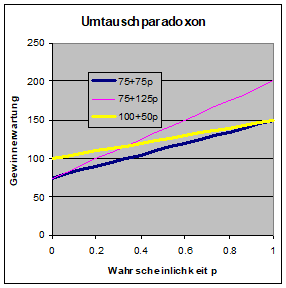tendency towards conciseness.

Addition 1. Let us assume that only two cases can be distinguished. The envelopes contain € 50 and € 100 in the first case and € 100 and € 200 in the second case. The person who fills the envelopes and offers them may like with the probability p Realize case 2 and otherwise case 1. Let us first assume that I am left completely in the dark about the contents of the envelopes. The content of the envelope I selected initially has no influence on my decision. I can choose not to swap in principle. That gives a profit expectation of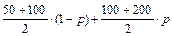€. That's 75 + 75p €. I get the same value if I choose the strategy of always swapping. As expected, the exchange does not improve anything.

Things look different if the person informs me in advance about the possible cases: Now I make my decision based on the content of the selected envelope: I always exchange when there is less than 200 € in the envelope. Now my profit expectation is 75 + 125p €. This strategy pays off so long p is greater than 0, so as long as the envelopes with the higher amounts come into question. Another variant is to only change if initially only € 50 has been drawn. The profit expectation is now 100 + 50p €. This strategy is superior to the one just described, so long p Is <1/3.

Addition 2. By including additional cases, these considerations can be easily generalized. There may even be (countable) an infinite number of cases. And an upper limit for the amount is by no means mandatory. For example, one could assign the probabilities ½, ¼, ⅛, ... to cases 1, 2, 3, .... The envelopes could then contain € 1 and € 2, € 2 and € 4, € 3 and € 6,…. This is only one of an immense variety of possible divisions. The best strategy for exchanging depends on what information is available about the cases and their probabilities.

The informed player will always swap under the conditions just described if he has initially drawn an odd euro value. Otherwise he sticks to his first choice. In this way he can improve his profit expectation by 5/9 € and thus reaches around 3 € and 56 cents instead of the 3 € for "blind" behavior.

(02.08.08)

My benefactor informs me that he did the following when filling the envelopes: initially he put € 1 in one envelope and € 2 in the other. Then he rolled the dice until he got the number 1 or 2. Whenever that was not the case, i.e. when the numbers were 3, 4, 5 or 6, he doubled the amounts in the envelopes. The result of this procedure - says the benefactor - is in the envelopes.

I pull an envelope and it's € 16 in it. I think about it: the probability of the case that this is the smaller amount has a ratio of 2: 3 to the probability of the other case. So I drew the smaller amount with the (conditional) probability 2/5 or 40% and the larger amount with the probability 3/5 or 60%. If I trade, the expected value is then 16 € multiplied by the factor 2 ∙ 40% + ½ ∙ 60%, that is 110%. The exchange makes you expect an average of 10% more than I hold in my hand. This corresponds to an average gain of € 1 and 60 cents. The risk of losing € 8 with a 60 percent chance is not that important. I think the swap is worthwhile. (The 10% gain expectation on exchange applies to every value drawn over € 1; for one euro it is equal to 100%.)

Contradiction. If I make the decision on the basis of the expected profit, I will always swap, no matter what amount I initially took. This results in the same contradiction as with the simple exchange paradox. Except that it is no longer so easy to dissolve.

Analysis. The proof that the always-swap strategy is superior to the never-swap strategy when the game is repeated and in the long term fails because the respective expected values ​​for the payouts are infinitely large. In fact, with a subjective assessment of the profit prospects and the risks, an exchange becomes more and more questionable, the higher the amount initially drawn. Incidentally, the risk argument also feeds considerable doubts about the offer and the sincerity of the benefactor, who does not have unlimited financial resources. We are faced with implementation difficulties similar to those with the pyramid scheme.

A detailed analysis can be found in Umtauschparadoxon.pdf. There is also a small stochastic simulation as an executable Java archive (Umtauschparadoxon.jar).

I took the reference to the difficult exchange paradox from the English language Wikipedia: http://en.wikipedia.org/wiki/Two_envelopes_problem.

(13.07.09)

Back to overview

### Semicircle

Task. Estimate the probability that three points of a circle, chosen purely randomly and independently of one another, lie on a - appropriately positioned - semicircle?

Contradiction. The probability is mostly underestimated - it is assumed to be below 50% - sometimes even 25% and less. With a small experiment you can convince yourself that the probability is much higher. It is exactly 75%.

Analysis. First you think of a fixed semicircle and conclude from this that there is a small probability that the three selected points lie on this semicircle. So the initial guess is about 1/8. Then you turn the semicircle in your mind so that as many of the points as possible are captured. Due to the possibility of adapting the semicircle to the position of the points, the probability must be greater than the initial estimate. The estimated value is corrected accordingly. This correction is done too cautiously (anchoring): “In manysituations, peoplemakeestimatesbystartingfrom an initial valuethatisadjustedtoyieldthefinalanswer ... In either case, adjustments are typically insufficient. That is, different starting points yield different estimates, which are biased toward the initial values ​​”(Tversky, Kahnemann, 1974).

(23.12.99)

Back to overview

In some cases, an action causes exactly the opposite of what it is intended to do (Spektrum der Wissenschaft 1992, Issue 11, pp. 23-26): An additional relief road makes the traffic jams worse (Dietrich Braess). To show how something like this can happen, I came up with the following game (it's a simplified version of the traffic problem):

It is a two-person game. The game master presents a drawing of a rectangle. Each player has the task of looking for a path along the edges of this rectangle that will lead them from the upper left corner to the lower right corner, at the lowest possible cost. The path costs are 2 units for each of the horizontal and 5 units for each of the vertical edges. If one of the edges is chosen by both players, they have to pay double the price due to mutual hindrance. One of the players chooses his route "around the top" and pays the route cost 7 (= 2 + 5). The other goes "around below" and also pays 7 (= 5 + 2) units.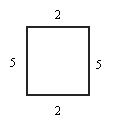The game master now gives players the opportunity to reduce their costs by introducing an additional and free connection from the top right to the bottom left. One of the players actually makes use of this possibility. He now only pays 6 (= 2 + 0 + 2 × 2) units.Since one of the connections is used by both, the other player now has to pay more, namely 9 (= 5 + 2 × 2) units. That doesn't leave him in peace, and he does it like his opponent.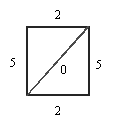Both finally choose the z-shaped path and each pay 8 (= 2 × 2 + 2 × 0 + 2 × 2) units. Although both now drive worse than at the beginning, when the relief connection did not yet exist, each of the players can only deviate from the z-shaped path to their own disadvantage. In game theory, something like this is called a Nash equilibrium. By the way: It doesn't help if both agree and take turns using the more advantageous route. The mean value is then still higher than for the separate routes. Both of them can only get out of this predicament if they decide to ignore the relief path and return to their original ways. That is where the paradox lies.

We are dealing here with a variant of the prisoner's dilemma that is dealt with extensively in “The Evolution of Cooperation” by Robert Axelrod (1987).

A mechanical analogue of the